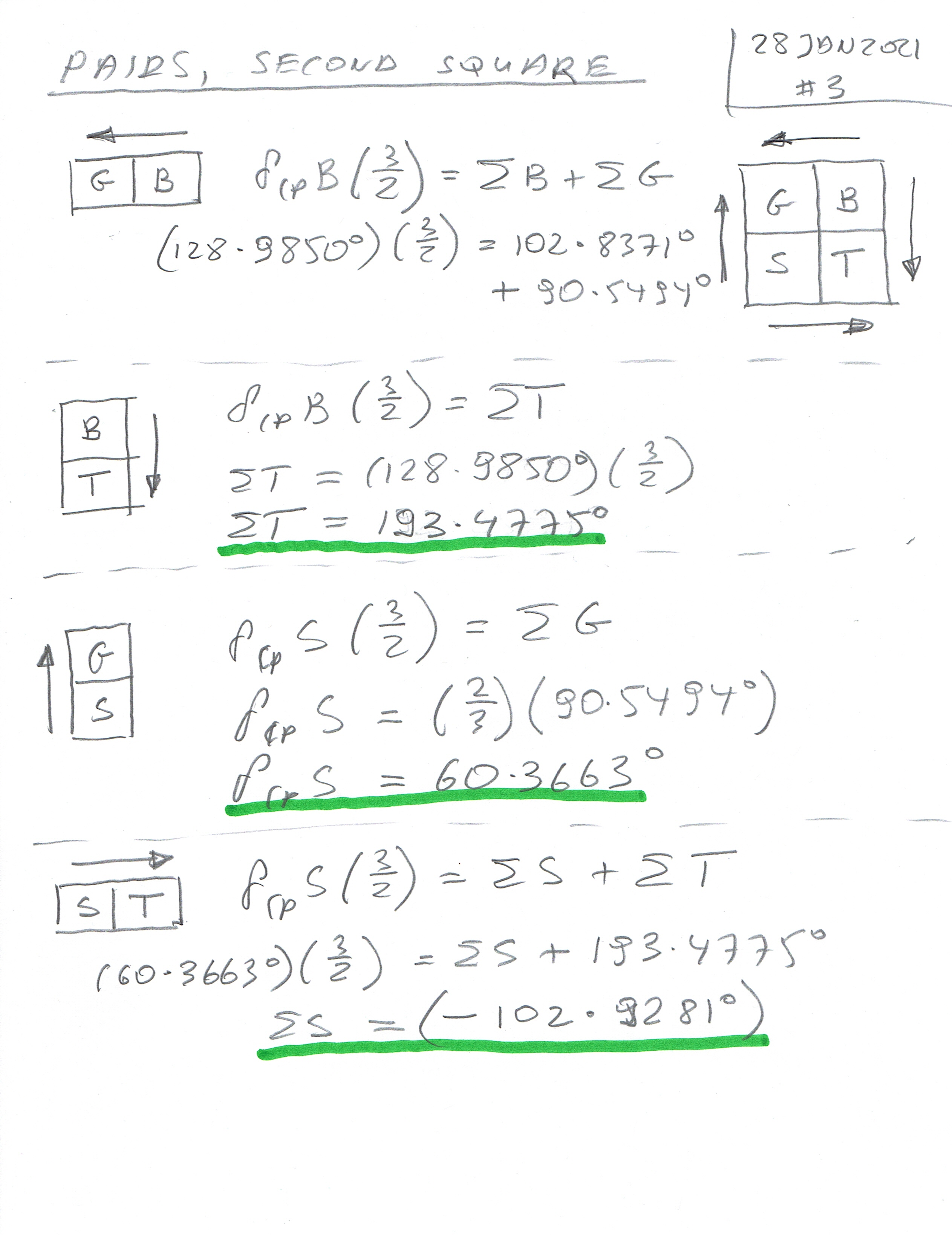Transfiguration of Our Lord; St Sixtus II (258 AD); St Felicissimus (258 AD); St Agapitus (258)

The eight elements of the Universe and Quantum Mechanics (Neutrino, Graviton, Space and Membrane Internal, and Quark, Boson, Time and Membrane External) can be divided into three squares, each consisting of four pairs. These pairs of elements relate Delta CP Violating Angles and Sigma Angles (sums of the Mixing Angles).

In this article, we will deal with two squares, giving altogether eight pairs of the elements.

The first square gives the following pairs:

Neutrino & Quark

Boson & Graviton

Quark and Boson

Graviton and Neutrino.

Fortunately, Neutrino and Quark angle values are known from the experiments. So the first pair gives us a 100% proven relation, which is:

(Delta CP Neutrino) * ( 3/2) = (Sigma Neutrino) + (Sigma Quark)

Now, Graviton and Boson were calculated before, so we have the mix of the elements known and unknown (50% known, 50% unknown). However, by analogy to the first equation ((Delta CP Neutrino) * ( 3/2) = (Sigma Neutrino) + (Sigma Quark)) we will have the following second equation:

(Delta CP Boson) * (3/2) = (Sigma Boson) + (Sigma Graviton)

This equation will allow us to test the results calculated from two simpler equations:

(Delta CP Quark) * (3/2) = (Sigma Boson)

and

(Delta CP Graviton) * (3/2) = (Sigma Neutrino)

All the pairs will follow this system of equations.

As you have noticed the coefficient factor is (3/2). I tried other coefficients such as 5/2, 7/2, 9/2, and 11/2 and their mix, but it seems that the factor 3/2 only gives good results (there will be an article at the end of this series with simple tests, where Angles form arithmetic and geometric series) and only 3/2 factor allows that.

In the following scripts you can see the calculations of Delta and Sigma Angles for four pairs mentioned before and the following pairs from the second square:

Boson & Graviton

Boson & Time

Space & Graviton

Space & Time.